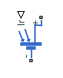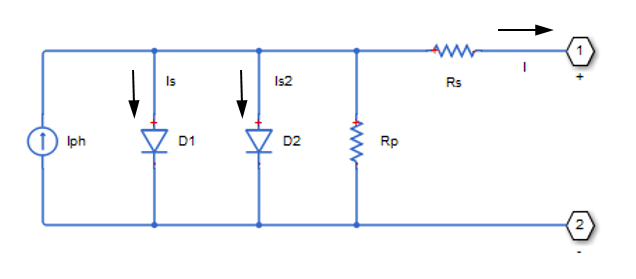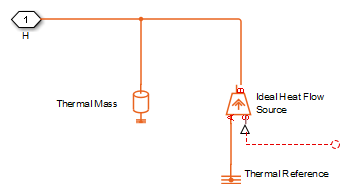# Solar Cell

Photovoltaic solar cell

•Libraries:
Simscape / Electrical / Sources

## Description

The Solar Cell block represents a solar cell current source.

The solar cell model includes the following components:

### Solar-Induced Current

The block represents a single solar cell as a resistance Rs that is connected in series with a parallel combination of the following elements:

• Current source

• Two exponential diodes

• Parallel resistor Rp

The following illustration shows the equivalent circuit diagram:The output current I is

`$I={I}_{ph}-{I}_{s}*\left({e}^{\left(V+I*{R}_{s}\right)/\left(\text{N}*{V}_{t}\right)}-1\right)-{I}_{s2}*\left({e}^{\left(V+I*{R}_{s}\right)/\left({N}_{2}*{V}_{t}\right)}-1\right)-\left(V+I*{R}_{s}\right)/{R}_{p}$`

where:

• Iph is the solar-induced current:

`${I}_{ph}={I}_{ph0}×\frac{{I}_{r}}{{I}_{r0}}$`

where:

• Ir is the irradiance (light intensity), in W/m2, falling on the cell.

• Iph0 is the measured solar-generated current for the irradiance Ir0.

• Is is the saturation current of the first diode.

• Is2 is the saturation current of the second diode.

• Vt is the thermal voltage, kT/q, where:

• k is the Boltzmann constant.

• T is the Device simulation temperature parameter value.

• q is the elementary charge on an electron.

• N is the quality factor (diode emission coefficient) of the first diode.

• N2 is the quality factor (diode emission coefficient) of the second diode.

• V is the voltage across the solar cell electrical ports.

The quality factor varies for amorphous cells, and is typically `2` for polycrystalline cells.

The block lets you choose between two models:

• An 8-parameter model where the preceding equation describes the output current

• A 5-parameter model that applies the following simplifying assumptions to the preceding equation:

• The saturation current of the second diode is zero.

• The impedance of the parallel resistor is infinite.

If you choose the 5-parameter model, you can parameterize this block in terms of the preceding equivalent circuit model parameters or in terms of the short-circuit current and open-circuit voltage the block uses to derive these parameters.

All models adjust the block resistance and current parameters as a function of temperature.

You can model any number of solar cells connected in series using a single Solar Cell block by setting the parameter Number of series-connected cells per string to a value larger than 1. Internally the block still simulates only the equations for a single solar cell, but scales up the output voltage according to the number of cells. This results in a more efficient simulation than if equations for each cell were simulated individually.

### Temperature Dependence

Several solar cell parameters depend on temperature. The solar cell temperature is specified by the Device simulation temperature parameter value.

The block provides the following relationship between the solar-induced current Iph and the solar cell temperature T:

`${I}_{ph}\left(T\right)={I}_{ph}*\left(1+TIPH1*\left(T-{T}_{meas}\right)\right)$`

where:

• TIPH1 is the First order temperature coefficient for Iph, TIPH1 parameter value.

• Tmeas is the Measurement temperature parameter value.

The block provides the following relationship between the saturation current of the first diode Is and the solar cell temperature T:

`${I}_{s}\left(T\right)={I}_{s}*{\left(\frac{T}{{T}_{meas}}\right)}^{\left(TXIS1}{N}\right)}*{e}^{\left(EG*\left(\frac{T}{{T}_{meas}}-1\right)/\left(N*{V}_{t}\right)\right)}$`

where TXIS1 is the Temperature exponent for Is, TXIS1 parameter value.

The block provides the following relationship between the saturation current of the second diode Is2 and the solar cell temperature T:

`${I}_{s2}\left(T\right)={I}_{s2}*{\left(\frac{T}{{T}_{meas}}\right)}^{\left(TXIS2}{{N}_{2}}\right)}*{e}^{\left(EG*\left(\frac{T}{{T}_{meas}}-1\right)/\left({N}_{2}*{V}_{t}\right)\right)}$`

where TXIS2 is the Temperature exponent for Is2, TXIS2 parameter value.

The block provides the following relationship between the series resistance Rs and the solar cell temperature T:

`${R}_{s}\left(T\right)={R}_{s}*{\left(\frac{T}{{T}_{meas}}\right)}^{TRS1}$`

where TRS1 is the Temperature exponent for Rs, TRS1 parameter value.

The block provides the following relationship between the parallel resistance Rp and the solar cell temperature T:

`${R}_{p}\left(T\right)={R}_{p}*{\left(\frac{T}{{T}_{meas}}\right)}^{TRP1}$`

where TRP1 is the Temperature exponent for Rp, TRP1 parameter value.

### Predefined Parameterization

There are multiple available built-in parameterizations for the Solar Cell block.

This pre-parameterization data allows you to set up the block to represent components by specific suppliers. The parameterizations of these solar cell modules match the manufacturer data sheets. To load a predefined parameterization, double-click the Solar Cell block, click the <click to select> hyperlink of the Selected part parameter and, in the Block Parameterization Manager window, select the part you want to use from the list of available components.

Note

The predefined parameterizations of Simscape™ components use available data sources for the parameter values. Engineering judgement and simplifying assumptions are used to fill in for missing data. As a result, expect deviations between simulated and actual physical behavior. To ensure accuracy, validate the simulated behavior against experimental data and refine component models as necessary.

For more information about pre-parameterization and for a list of the available components, see List of Pre-Parameterized Components.

### Thermal Port

You can expose the thermal port to model the effects of generated heat and device temperature. To expose the thermal port, set the Modeling option parameter to either:

• `No thermal port` — The block does not contain a thermal port and does not simulate heat generation in the device.

• `Show thermal port` — The block contains a thermal port that allows you to model the heat that conduction losses generate. For numerical efficiency, the thermal state does not affect the electrical behavior of the block.

For more information on using thermal ports and on the Thermal Port parameters, see Simulating Thermal Effects in Semiconductors.

The thermal port model, shown in the following illustration, represents just the thermal mass of the device. The thermal mass is directly connected to the component thermal port H. An internal Ideal Heat Flow Source block supplies a heat flow to the port and thermal mass. This heat flow represents the internally generated heat.The internally generated heat in the solar cell is calculated according to the equivalent circuit diagram, shown at the beginning of the reference page, in the Solar-Induced Current section. It is the sum of the i2 · R losses for each of the resistors plus the losses in each of the diodes.

The internally generated heat due to electrical losses is a separate heating effect to that of the solar irradiation. To model thermal heating due to solar irradiation, you must account for it separately in your model and add the heat flow to the physical node connected to the solar cell thermal port.

## Ports

### Input

expand all

Physical signal associated with the solar cell incident irradiance.

### Conserving

expand all

Electrical conserving port associated with the solar cell positive voltage

Electrical conserving port associated with the solar cell negative voltage

Thermal conserving port.

#### Dependencies

To enable this port, set Modeling option to `Show thermal port`.

## Parameters

expand all

Whether to parameterize the block to represent components by specific suppliers. Click the <click to select> hyperlink to open the Block Parameterization Manager window. For more information on the Block Parameterization Manager, see Load Predefined Parameterizations.

Whether to enable the thermal port of the block and model the effects of generated heat and device temperature.

### Cell Characteristics

All quantities in this setting are for a single cell.

Select one of the following methods for block parameterization:

• ```By s/c current and o/c voltage, 5 parameter``` — Provide short-circuit current and open-circuit voltage that the block converts to an equivalent circuit model of the solar cell.

• ```By equivalent circuit parameters, 5 parameter``` — Provide electrical parameters for an equivalent circuit model of the solar cell using the 5-parameter solar cell model that makes the following assumptions:

• The saturation current of the second diode is zero.

• The parallel resistor has infinite impedance.

• ```By equivalent circuit parameters, 8 parameter``` — Provide electrical parameters for an equivalent circuit model of the solar cell using the 8-parameter solar cell model.

Current that flows when you short-circuit the solar cell.

#### Dependencies

This parameter is visible only when you select ```By s/c current and o/c voltage, 5 parameter``` for the Parameterize by parameter.

Voltage across the solar cell when it is not connected.

#### Dependencies

This parameter is visible only when you select ```By s/c current and o/c voltage, 5 parameter``` for the Parameterize by parameter.

Asymptotic reverse current of the first diode for increasing reverse bias in the absence of any incident light.

#### Dependencies

This parameter is visible only when you select ```By equivalent circuit parameters, 5 parameter``` or ```By equivalent circuit parameters, 8 parameter``` for the Parameterize by parameter.

Asymptotic reverse current of the second diode for increasing reverse bias in the absence of any incident light.

#### Dependencies

This parameter is visible only when you select ```By equivalent circuit parameters, 8 parameter``` for the Parameterize by parameter.

Solar-induced current when the irradiance is Ir0.

#### Dependencies

This parameter is visible only when you select ```By equivalent circuit parameters, 5 parameter``` or ```By equivalent circuit parameters, 8 parameter``` for the Parameterize by parameter.

Irradiance that produces a current of Iph0 in the solar cell.

Emission coefficient of the first diode.

Emission coefficient of the second diode.

#### Dependencies

This parameter is visible only when you select ```By equivalent circuit parameters, 8 parameter``` for the Parameterize by parameter.

Internal series resistance.

Internal parallel resistance.

#### Dependencies

This parameter is visible only when you select ```By equivalent circuit parameters, 8 parameter``` for the Parameterize by parameter.

### Panel Configuration

Number of series-connected solar cells per string modeled by the block. The value must be greater than `0`.

Number of strings connected in parallel. The value must be greater than `0`.

### Temperature Dependence

Order of the linear increase in the solar-generated current as temperature increases. The value must be greater than or equal to `0`.

Solar cell activation energy. The value must be greater than or equal to 0.1.

Order of the exponential increase in the current from the first diode as temperature increases. The value must be greater than `0`.

Order of the exponential increase in the current from the second diode as temperature increases. The value must be greater than or equal to `0`.

#### Dependencies

This parameter is visible only when you select ```By equivalent circuit parameters, 8 parameter``` for the Parameterize by parameter.

Order of the exponential increase in the series resistance as temperature increases. The value must be greater than or equal to `0`.

Order of the exponential increase in the parallel resistance as temperature increases. The value must be greater than or equal to `0`.

#### Dependencies

This parameter is visible only when you select ```By equivalent circuit parameters, 8 parameter``` for the Parameterize by parameter.

Temperature at which the solar cell parameters were measured. The value must be greater than `0`.

Temperature at which the solar cell is simulated. The value must be greater than `0`.

### Thermal port

To enable these parameters, set Modeling option to `Show thermal port`.

The heat energy required to raise the temperature of one solar cell by one degree. When modeling more than one cell in series, specify the thermal mass for a single cell. This value gets multiplied internally by the number of cells to determine the total thermal mass.

The temperature of the solar cell at the start of simulation.

 Gow, J.A. and C.D. Manning. “Development of a Photovoltaic Array Model for Use in Power-Electronics Simulation Studies.” IEEE Proceedings of Electric Power Applications, Vol. 146, No. 2, 1999, pp. 193–200.